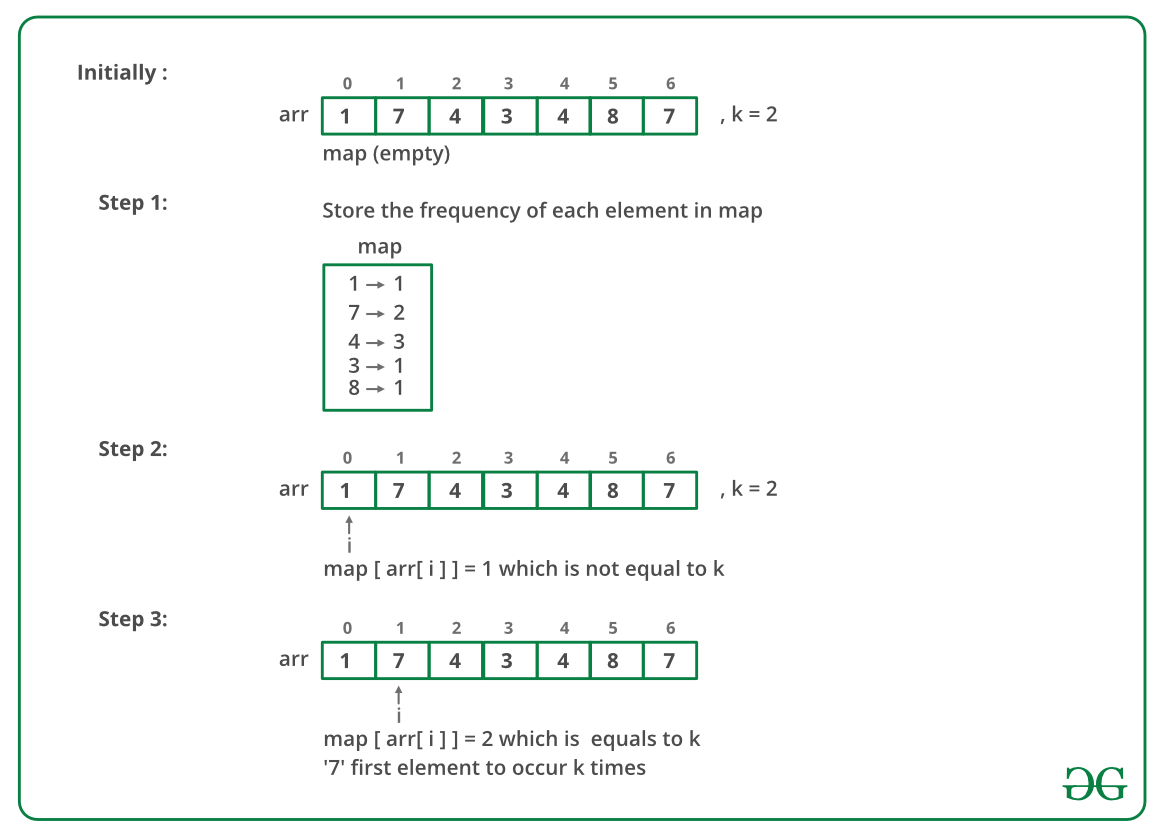Related Articles

# First element occurring k times in an array

• Difficulty Level : Easy
• Last Updated : 16 Aug, 2021

Given an array of n integers. The task is to find the first element that occurs k number of times. If no element occurs k times the print -1. The distribution of integer elements could be in any range.
Examples:

Input: {1, 7, 4, 3, 4, 8, 7},
k = 2
Output: 7
Both 7 and 4 occur 2 times.
But 7 is the first that occurs 2 times.

Input: {4, 1, 6, 1, 6, 4},
k = 1
Output: -1

Simple Approach: By using two loops, count the number of times a number appears in the array.
Time complexity: O(n2).
Efficient Approach: Use unordered_map for hashing as range is not known. Steps:

1. Traverse the array elements from left to right.
2. While traversing increment their count in the hash table.
3. Again traverse the array from left to right and check which element has a count equal to k. Print that element and stop.
4. If no element has a count equal to k, print -1.

Below is a dry run of the above approach:Below is the implementation of the above approach:

## C++

 `// C++ implementation to find first``// element occurring k times``#include ` `using` `namespace` `std;` `// function to find the first element``// occurring k number of times``int` `firstElement(``int` `arr[], ``int` `n, ``int` `k)``{``    ``// unordered_map to count``    ``// occurrences of each element``    ``unordered_map<``int``, ``int``> count_map;``    ``for` `(``int` `i=0; i

## Java

 `import` `java.util.HashMap;` `// Java implementation to find first``// element occurring k times``class` `GFG {` `// function to find the first element``// occurring k number of times``    ``static` `int` `firstElement(``int` `arr[], ``int` `n, ``int` `k) {``        ``// unordered_map to count``        ``// occurrences of each element` `        ``HashMap count_map = ``new` `HashMap<>();``        ``for` `(``int` `i = ``0``; i < n; i++) {``            ``int` `a = ``0``;``            ``if``(count_map.get(arr[i])!=``null``){``                ``a = count_map.get(arr[i]);``            ``}``            ` `            ``count_map.put(arr[i], a+``1``);``        ``}``        ``//count_map[arr[i]]++;` `        ``for` `(``int` `i = ``0``; i < n; i++) ``// if count of element == k ,then``        ``// it is the required first element``        ``{``            ``if` `(count_map.get(arr[i]) == k) {``                ``return` `arr[i];``            ``}``        ``}` `        ``// no element occurs k times``        ``return` `-``1``;``    ``}` `// Driver program to test above``    ``public` `static` `void` `main(String[] args) {``        ``int` `arr[] = {``1``, ``7``, ``4``, ``3``, ``4``, ``8``, ``7``};``        ``int` `n = arr.length;``        ``int` `k = ``2``;``        ``System.out.println(firstElement(arr, n, k));``    ``}``}` `//this code contributed by Rajput-Ji`

## Python3

 `# Python3 implementation to``# find first element``# occurring k times` `# function to find the``# first element occurring``# k number of times``def` `firstElement(arr, n, k):` `    ``# dictionary to count``    ``# occurrences of``    ``# each element``    ``count_map ``=` `{};``    ``for` `i ``in` `range``(``0``, n):``        ``if``(arr[i] ``in` `count_map.keys()):``            ``count_map[arr[i]] ``+``=` `1``        ``else``:``            ``count_map[arr[i]] ``=` `1``        ``i ``+``=` `1``    ` `    ``for` `i ``in` `range``(``0``, n):``        ` `        ``# if count of element == k ,``        ``# then it is the required``        ``# first element``        ``if` `(count_map[arr[i]] ``=``=` `k):``            ``return` `arr[i]``        ``i ``+``=` `1``            ` `    ``# no element occurs k times``    ``return` `-``1` `# Driver Code``if` `__name__``=``=``"__main__"``:` `    ``arr ``=` `[``1``, ``7``, ``4``, ``3``, ``4``, ``8``, ``7``];``    ``n ``=` `len``(arr)``    ``k ``=` `2``    ``print``(firstElement(arr, n, k))` `# This code is contributed``# by Abhishek Sharma`

## C#

 `// C# implementation to find first``// element occurring k times``using` `System;``using` `System.Collections.Generic;` `class` `GFG``{` `    ``// function to find the first element``    ``// occurring k number of times``    ``static` `int` `firstElement(``int` `[]arr, ``int` `n, ``int` `k)``    ``{``        ``// unordered_map to count``        ``// occurrences of each element` `        ``Dictionary<``int``, ``int``> count_map = ``new` `Dictionary<``int``,``int``>();``        ``for` `(``int` `i = 0; i < n; i++)``        ``{``            ``int` `a = 0;``            ``if``(count_map.ContainsKey(arr[i]))``            ``{``                ``a = count_map[arr[i]];``                ``count_map.Remove(arr[i]);``                ``count_map.Add(arr[i], a+1);``            ``}``            ``else``                ``count_map.Add(arr[i], 1);``        ``}``        ``//count_map[arr[i]]++;` `        ``for` `(``int` `i = 0; i < n; i++) ``// if count of element == k ,then``        ``// it is the required first element``        ``{``            ``if` `(count_map[arr[i]] == k)``            ``{``                ``return` `arr[i];``            ``}``        ``}` `        ``// no element occurs k times``        ``return` `-1;``    ``}` `    ``// Driver code``    ``public` `static` `void` `Main(String[] args)``    ``{``        ``int` `[]arr = {1, 7, 4, 3, 4, 8, 7};``        ``int` `n = arr.Length;``        ``int` `k = 2;``        ``Console.WriteLine(firstElement(arr, n, k));``    ``}``}` `// This code has been contributed by 29AjayKumar`

## Javascript

 `

Output:

`7`

Time Complexity: O(n)
This article is contributed by Ayush Jauhari. If you like GeeksforGeeks and would like to contribute, you can also write an article using write.geeksforgeeks.org or mail your article to review-team@geeksforgeeks.org. See your article appearing on the GeeksforGeeks main page and help other Geeks.

#### Method 3:Using Built-in Python functions:

• Count the frequencies of every element using Counter function
• Traverse in frequency dictionary
• check which element has a count equal to k. Print that element and stop.
• If no element has a count equal to k, print -1.

## Python3

 `# importing counter from collections``from` `collections ``import` `Counter` `# Python3 implementation to find``# first element occurring k times``# function to find the  first element``# occurring  k number of times``def` `firstElement(arr, n, k):` `    ``# calculating frequencies usinf Counter``    ``count_map ``=` `Counter(arr)` `    ``for` `i ``in` `range``(``0``, n):` `        ``# If count of element == k ,``        ``# then it is the required``        ``# first element``        ``if` `(count_map[arr[i]] ``=``=` `k):``            ``return` `arr[i]``        ``i ``+``=` `1` `    ``# No element occurs k times``    ``return` `-``1`  `# Driver Code``if` `__name__ ``=``=` `"__main__"``:` `    ``arr ``=` `[``1``, ``7``, ``4``, ``3``, ``4``, ``8``, ``7``]``    ``n ``=` `len``(arr)``    ``k ``=` `2``    ``print``(firstElement(arr, n, k))` `# This code is contributed by vikkycirus`

Output:

`7`

Time Complexity: O(N)

Attention reader! Don’t stop learning now. Get hold of all the important DSA concepts with the DSA Self Paced Course at a student-friendly price and become industry ready.  To complete your preparation from learning a language to DS Algo and many more,  please refer Complete Interview Preparation Course.

In case you wish to attend live classes with experts, please refer DSA Live Classes for Working Professionals and Competitive Programming Live for Students.

My Personal Notes arrow_drop_up